﻿ 车载摄像头脏污识别方法 Methods of Vehicle Camera Dirty Identification

Journal of Image and Signal Processing
Vol. 09  No. 01 ( 2020 ), Article ID: 33284 , 10 pages
10.12677/JISP.2020.91002

Methods of Vehicle Camera Dirty Identification

Yu Gao, Zhijun Wu

School of Automotive Studies, Tongji University, Shanghai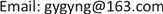Received: Nov. 12th, 2019; accepted: Nov. 27th, 2019; published: Dec. 4th, 2019ABSTRACT

The degradation of image quality caused by dirty in lens surface will reduce the accuracy of image recognition algorithm, which eventually has a great impact on the driving safety of intelligent driving assistance system (IDAS). Images captured on the road are no reference images. In this article, methods of no reference image quality assessment such as correlation coefficient, edge information, frequency domain energy and average information entropy will be used in the image recognition. Those methods are used to distinguish the clear images and dirty images obtained on the road. The results show that those methods have a certain degree of distinction between clear images and dirty images.

Keywords:IDAS, No Reference, Image Quality, Definition1. 引言

2. 图像获取及预处理

2.1. 实验图像获取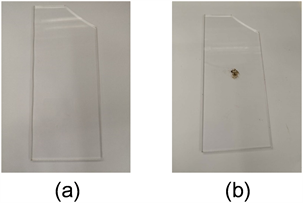Figure 1. Acrylic plate placed in front of the camera: (a) Clear acrylic plate; (b) Dirty acrylic plate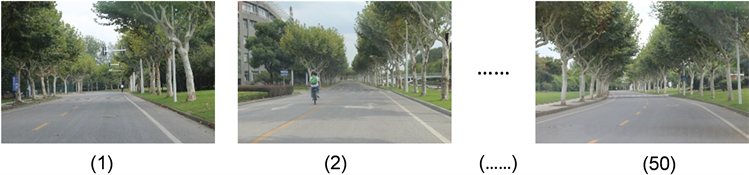Figure 2. Clear image sequence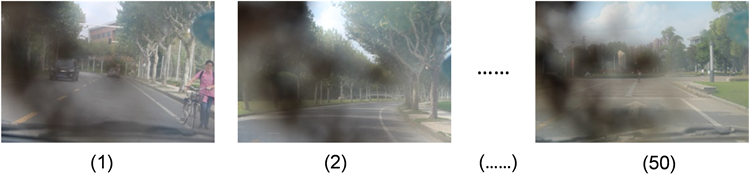Figure 3. Dirty image sequence

2.2. 图像预处理

3. 算法实现

3.1. 图像相关性检测

${r}_{k}=\frac{{\sum }_{m}{\sum }_{n}\left({A}_{k,mn}-\stackrel{¯}{{A}_{k}}\right)\left({A}_{k-1,mn}-\stackrel{¯}{{A}_{k-1}}\right)}{\sqrt{\left({{\sum }_{m}{\sum }_{n}\left({A}_{k,mn}-\stackrel{¯}{{A}_{k}}\right)}^{2}\right)\left({{\sum }_{m}{\sum }_{n}\left({A}_{k-1,mn}-\stackrel{¯}{{A}_{k-1}}\right)}^{2}\right)}}\stackrel{¯}{A}$ (1)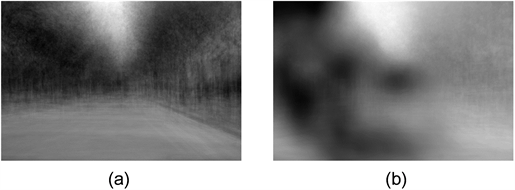Figure 4. Average pixel image: (a) Clear image sequence; (b) Dirty image sequence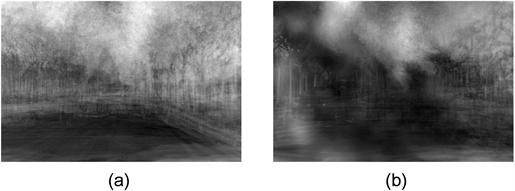Figure 5. Pixel standard deviation image: (a) Clear image sequence; (b) Dirty image sequence

${r}_{k}=\frac{{\sum }_{m}{\sum }_{n}\left({A}_{k,mn}-\stackrel{¯}{{A}_{k}}\right)\left({A}_{k-1,mn}-\stackrel{¯}{{A}_{k-1}}\right)}{\sqrt{\left({{\sum }_{m}{\sum }_{n}\left({A}_{k,mn}-\stackrel{¯}{{A}_{k}}\right)}^{2}\right)\left({{\sum }_{m}{\sum }_{n}\left({A}_{k-1,mn}-\stackrel{¯}{{A}_{k-1}}\right)}^{2}\right)}}$ (2)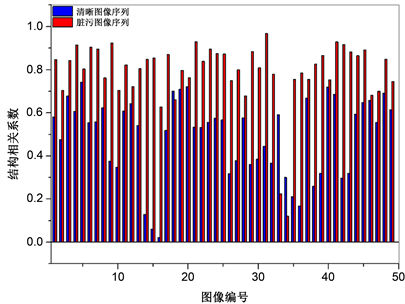Figure 6. Image structure correlation coefficient

3.2. 图像边缘信息Figure 7. Image edge: (a) Clear image sequence; (b) Dirty image sequence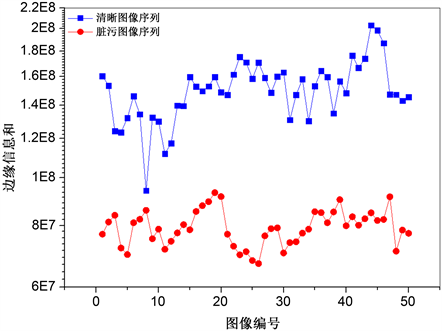Figure 8. Edge information comparison of different image sequences

3.3. 图像频域能量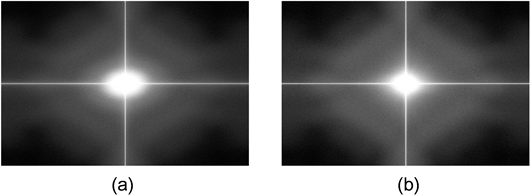Figure 9. Image spectral distribution: (a) Clear image sequence; (b) Dirty image sequence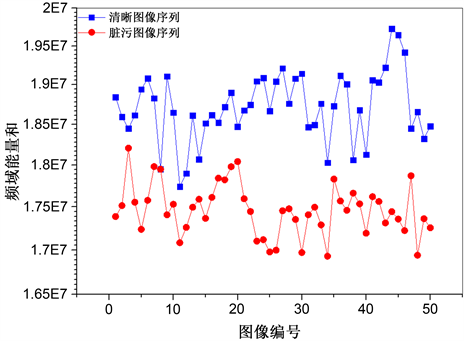Figure 10. Frequency domain energy of different image sequences

3.4. 图像信息熵

$H=-\underset{k=1}{\overset{256}{\sum }}p\left(k\right)×{\mathrm{log}}_{2}p\left(k\right)$ (3)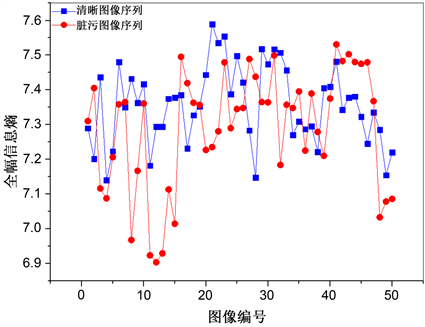Figure 11. Global information entropy of different image sequences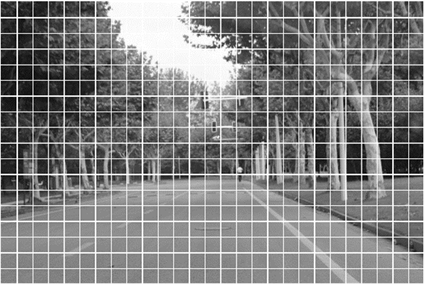Figure 12. Zone division mode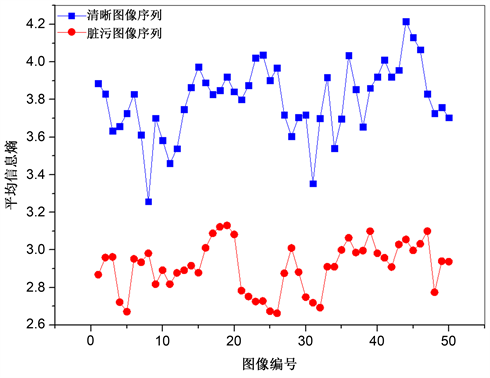Figure 13. Average information entropy of different image sequences

4. 结论

1) 使用相关系数法、边缘信息、频域能量和平均信息熵都可以将清晰图像序列和脏污图像序列区分开。脏污图像序列中的图像相关性大于清晰图像序列中的图像相关性；清晰图像序列中的边缘信息高于脏污图像序列中的边缘信息；清晰图像序列中的频域能量高于脏污图像序列中的频域能量；清晰图像序列的平均信息熵高于脏污图像序列的平均信息熵。

2) 在图像尺寸相同的情况下，平均信息熵计算速度最快，有较高的应用价值。

Methods of Vehicle Camera Dirty Identification[J]. 图像与信号处理, 2020, 09(01): 8-17. https://doi.org/10.12677/JISP.2020.91002

1. 1. 牛欣宇. 混合失真图像的无参考质量评价方法研究[D]: [硕士学位论文]. 哈尔滨: 哈尔滨工业大学, 2017.

2. 2. 李俊峰, 张飞燕, 戴文战, 等. 基于图像相关性和结构信息的无参考图像质量评价[J]. 光电子·激光, 2014(12): 2407-2416.

3. 3. Gu, J., Belhumeur, P., Nayar, S., et al. (2009) Removing Image Artifacts Due to Dirty Camera Lenses and Thin Occluders. ACM Transactions on Graphics, 28, 1-10.
https://doi.org/10.1145/1618452.1618490

4. 4. Fujii, Y., Ohta, N., Ito, T., et al. (2006) Image Restoration for Security Cameras with Dirty Lens under Oblique Illumination. IEEE International Workshop on Imagining Systems & Techniques.

5. 5. Einecke, N., Gandhi, H. and Jorg, D. (2014) Detection of Camera Artifacts from Camera Images. IEEE International Conference on Intelligent Transportation Systems, Qingdao, 8-11 October 2014, 603-610.
https://doi.org/10.1109/ITSC.2014.6957756

6. 6. 王春哲, 李杰. 基于边缘信息的图像质量评价研究[J]. 长春大学学报(自然科学版), 2014(5): 1330-1333.

7. 7. 田若良, 刘柏森. 基于频域能量分割的图像模糊度评价方法[J]. 计算机技术与发展, 2015(6): 101-105.

8. 8. 郑加苏. 基于图像信息熵的无参考图像质量评估算法的研究[D]: [硕士学位论文]. 北京: 北京交通大学, 2015.